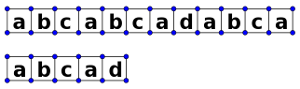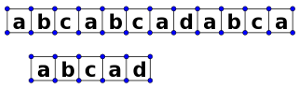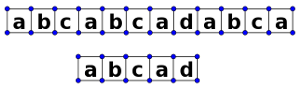## 引言和预备定义

int Match_String(char *s, char *t) {
//在主串中匹配子串，并返回子串在主串中的位置
int i = 0, j = 0;
while(s[i] && t[j]) {
if (s[i] == t[j])
++i, ++j;//比较下一个字符
else
i = i - j + 1, j = 0;//i退回到下一个字符，j归零
}
if (j == strlen(t))
return i - j;//返回子串首字符对应的位置
else
return -1;//这里-1表示不匹配
}## 算法主体

next(j) =

• k，k是一个最大的s，能从子串的下标为j-1的字符开始，向前找一个长为s的序列，它和子串开头s个字符相等。
• 0，j = 1或者如果找不到上述的s；（这里就是向前迈进一步的意思）

int KMP(char *s, char *t) {
int i = 0, j = 0;
while (s[i] && t[j]) {
if (j == 0 || s[i] == t[j])
++i, ++j;//比较下一个字符
else
j = next(j);//比较第next(j)个字符
}
if (j == strlen(t))
return i - j;//返回子串首字符对应的位置
else
return -1;//这里-1表示不匹配
}


## next(j)函数

• 当j = 1时，只有t = s[i-1] = t[j-1]，那直接用t去和s[i]比就行了，所以next(1) = 1。
• 假设next(j) = n，即t～t[n-1]和t[j-n]～t[j-1]相等，那么考虑next(j + 1)时的情形：
1. 若t[n] = t[j]，则显然我们找到了一个更长的匹配列:t～t[n]和t[j-n]～t[j]相等，next(j + 1) = next(j) + 1;
2. 若t[n] ≠ t[j]，那么我们得从新考虑寻找子串开头与结尾的最长匹配列的问题，但是我们再一看，t[n] ≠ t[j]，这不就是一个“失配”问题吗？好比从t与t[j-n]开始配对，直到t[n-1]于t[j-1]，但是在第n位上发生失配，这时该怎么办？按照我们上一节的讨论，应该寻求一个next(n)位来于t[j]位相配，这个时候，问题就变成递归性质的了，如果t[next(n)] = t[j]，那么问题变成了第一条。如果t[next(n)] ≠ t[j]，那么问题变成了第二条。

void get_next(char *t, int *next) {
int i = 1, j = 0;
next = 0;
while(t[i]) {
if (j == 0 || t[i - 1] == t[j - 1]) {//第一种情况
++i, ++j;
next[i] = j;
}
else
j = next[j];//第二种情况，直到相等为止才会进入上一个分支
}
}


int KMP(char *s, char *t, int *next) {
int i = 0, j = 0;
while (s[i] && t[j]) {
if (j == 0 || s[i] == t[j])
++i, ++j;//比较下一个字符
else
j = next[j];//比较第next(j)个字符
}
if (j == strlen(t))
return i - j;//返回子串首字符对应的位置
else
return -1;//这里-1表示不匹配
}


## 总结

1. next(j)的求法。要点是把寻找匹配序列的时候，把整个问题也当做一个“失配问题”，自身既是主串也是子串。
2. 代码实现时的下标问题。这个问题我在第一次自己写的时候也出现了很多问题，当然代码的实现自然有很多种，我这里就说我自己写的这种吧。一方面是实现next()的时候要想到用数组储存之前的值，否则你的递归会很麻烦。另一方面是实现next的比较过程，我用了t[i - 1] == t[j - 1]，事实上比较确实可以从第一个开始，无需管第零个，但是这里涉及到存放next[j]数组的问题，所以考虑好下标后从这么写的。